What's newBig square, tiny envelope

By David, 20 March 2017 Activity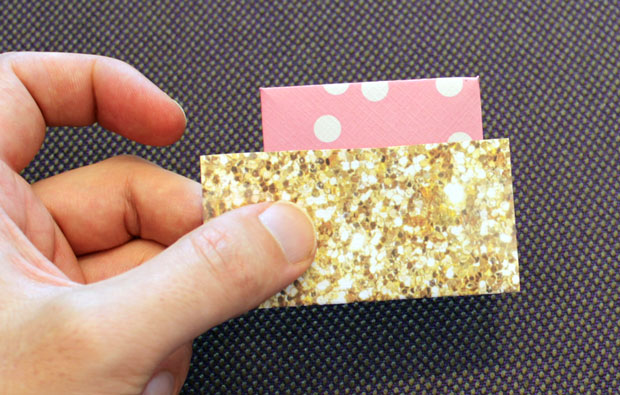Can you fit the pink square into the brown envelope?

Here’s a quick puzzle for you – this square is too wide for its envelope. Can you find a way to make it fit?

You will need

• Two equal squares of paper
• Sticky tape

Preparation

1. Fold one of the squares in half to make a rectangle.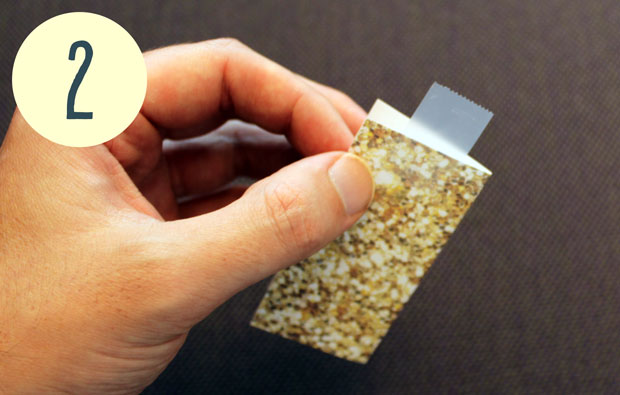Put tape around both ends of the rectangle.

2. Put a piece of sticky tape around one short edge of the rectangle, and a second piece around the other, sealing the ends shut. You should have a pouch or envelope, with one open side.
3. For the second square, mark the midpoints of each side. Then fold each corner of the square into the middle, making sure the crease runs from midpoint to midpoint.
4. You will end up with a square that doesn’t fit neatly in the envelope.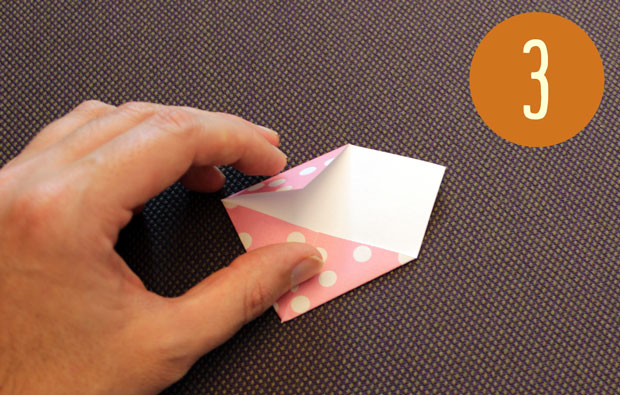Fold all the corners into the middle to make a square.

Challenge

1. The aim of this activity is to put the square fully inside the envelope, with no part peaking outside.
2. You can bend the square but you can’t crease it, and when you’re finished it has to lie flat.
3. You can’t break the sticky tape on the envelope.

What’s happening?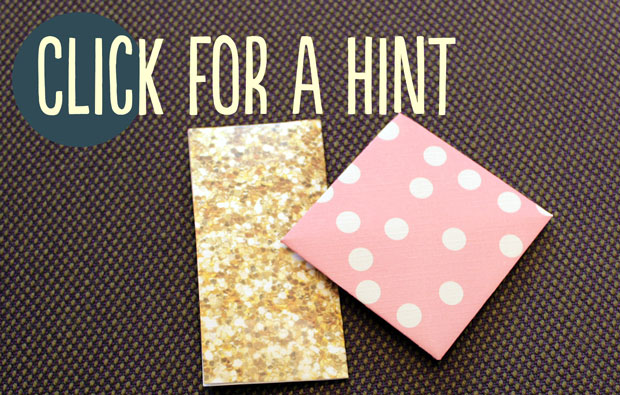This puzzle is a bit hard to explain in writing, but once you have solved it and know the answer, it’s really fun to try with your friends!

The main trick is realising that the square has to stay the same size and shape. If the square can’t change, then the envelope has to! (If you haven’t found the solution, this hint might help.)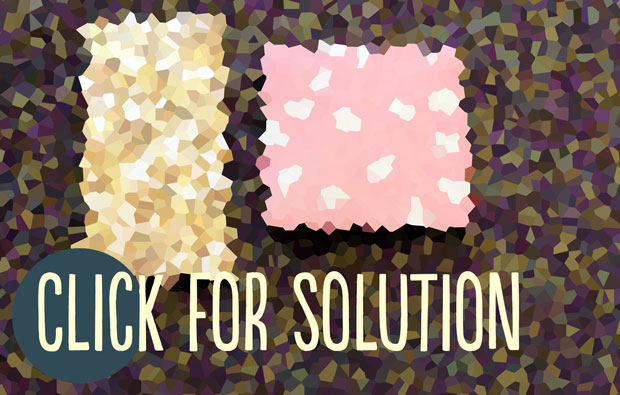The answer to the puzzle indirectly proves the rectangular envelope and the square you put inside it have the same area. If the short side of the rectangle is one inch long, the long side is two inches, giving it an area of two square inches. The square has sides that are about 1.41 inches long, and multiplying 1.41… by 1.41… gives an area of two square inches. The number 1.41… has another name – it’s the square root of two.

The square root of two is a number that pops up all the time. If you imagine shrinking a sheet of A4 paper down so the short side is one centimetre long, the longer side would be exactly the square root of two centimetres long!

Fast fact

In the early 14th century an inch was defined as “three grains of barley, dry and round, placed end to end lengthwise”! These days, the inch is actually based on the metric system. One inch is exactly 2.54 centimetres long!

If you’re after more hands-on activities for kids, subscribe to Double Helix magazine!Categories: